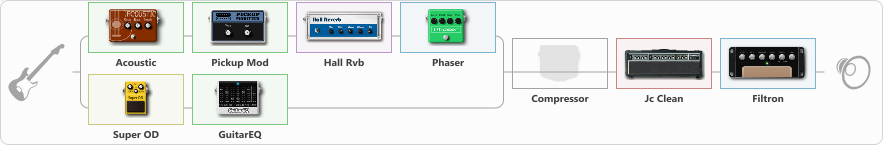# Dumb Shit

Discussion in 'ToneLib-GFX presets' started by lulZghost, Dec 2, 2021.

1. Dumb Shit

Preset name: Dumb Shit

I just threw some components together, randomly dialed the knobs and the result sounds like this.

Effects chain:Effect: "Splitter" (Dynamics / Filter)
{
"A-Bypass" = Off
"A-Pan" = 0
"A-Level" = 55
"B-Bypass" = Off
"B-Pan" = 0
"B-Level" = 55

'A' branch:
{

Effect: "Acoustic" (Dynamics / Filter), active - "yes"
{
"Style" = Arpeggio/Classic
"Resonance" = 100
"Bass" = 55
"Treble" = 55
}

Effect: "Pickup Mod" (Dynamics / Filter), active - "yes"
{
"Mode" = Phase Out
"Tone" = 50
}

Effect: "Hall Rvb" (Reverberation), active - "yes"
{
"Time" = 1.7
"PreDelay" = 0
"LoDamp" = 0
"HiDamp" = 17
"Mix" = 44
}

Effect: "Phaser" (Modulation / Sfx), active - "yes"
{
"Model" = Boss PH-3
"Speed" = 5.0
"Depth" = 100
"Reso" = 26
"Freq" = 5.0
}
}
'B' branch:
{

Effect: "Super OD" (Overdrive / Distortion), active - "yes"
{
"Drive" = 8
"Tone" = 80
"Level" = 91
}

Effect: "GuitarEQ" (Dynamics / Filter), active - "yes"
{
"160 Hz" = 0
"400 Hz" = 0
"800 Hz" = 0
"1.6 kHz" = 0
"3.2 kHz" = 0
"6.4 kHz" = 0
"12 kHz" = 0
"Level (dB)" = 0
}
}
}

Effect: "Compressor" (Dynamics / Filter), active - "no"
{
"Sense" = 21
"Level" = 50
}

Effect: "Jc Clean" (Amp simulators), active - "yes"
{
"Gain" = 50
"Bass" = 50
"Middle" = 50
"Treble" = 50
"Presence" = 50
"Master" = 50
"Level (dB)" = 0
}

Effect: "Filtron" (Modulation / Sfx), active - "yes"
{
"Attack" = 2.7
"Depth" = 72
"Reso" = 60
"Cutoff" = 6.1
"Polarity" = Up
"Sens" = 50
}

Note: You will need to download and install the ToneLib-GFX software to use the preset.

#### Attached Files:

• ###### Dumb_Shit.tlgfx
File size:
932 bytes
Views:
2,076
4rerq1 likes this.